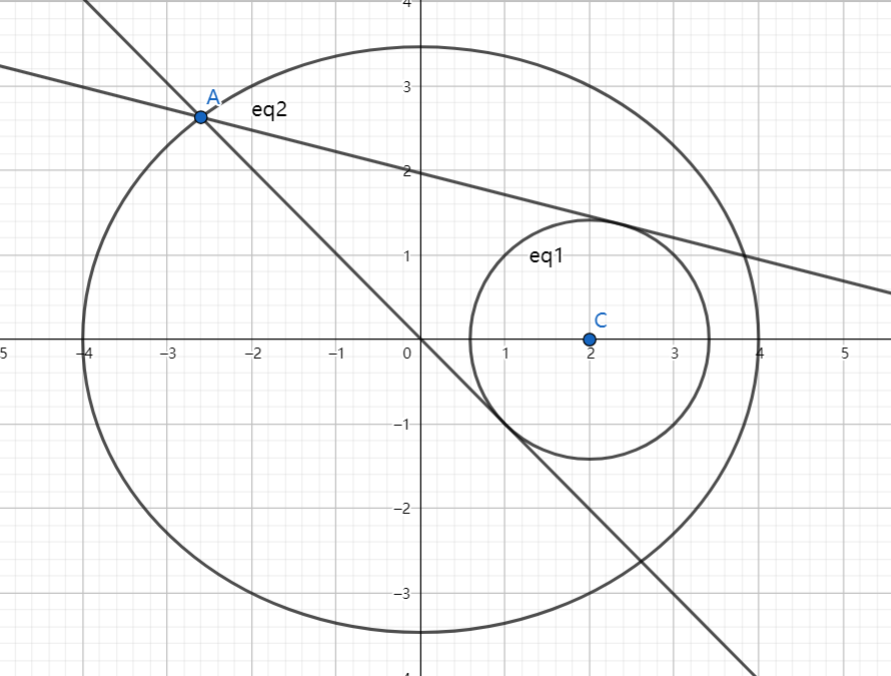# SAT1000 - P801

Geometry Level pendingAs shown above, the ellipse $E$ has equation: $\dfrac{x^2}{16}+\dfrac{y^2}{12}=1$, and point $C$ is the center of the circle: $x^2+y^2-4x+2=0$ If $P$ is a point on ellipse $E$, $l_1, l_2$ both pass through $P$ and they are both tangent to circle $C$, and the product of the slope of $l_1, l_2$ is equal to $\dfrac{1}{2}$, then find the all possible coordinate(s) of point $P$.

How to submit:

• First, find the number of all possible solutions. Let $N$ denote the number of solutions.

• Then Sort the solutions by x-coordinate from smallest to largest, if the x-coordinate is the same, then sort by y-coordinate from smallest to largest.

• Let the sorted solutions be: $(x_1,y_1), (x_2,y_2), (x_3,y_3), \cdots ,(x_N,y_N)$, then $M=\displaystyle \sum_{k=1}^N k(x_k+y_k)$.

For instance, if the solution is: $(-1,2), (-1,1), (1,3), (0,4)$

Then the sorted solution will be: $(-1,1), (-1,2), (0,4), (1,3)$

Then $N=4$, $M=\displaystyle \sum_{k=1}^4 k(x_k+y_k)= 1 \times (-1+1) + 2 \times (-1+2) + 3 \times (0+4) + 4 \times (1+3) =30$.

For this problem, submit $\lfloor 1000(M+N) \rfloor$.

Have a look at my problem set: SAT 1000 problems

×